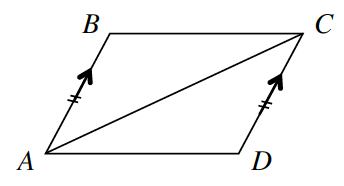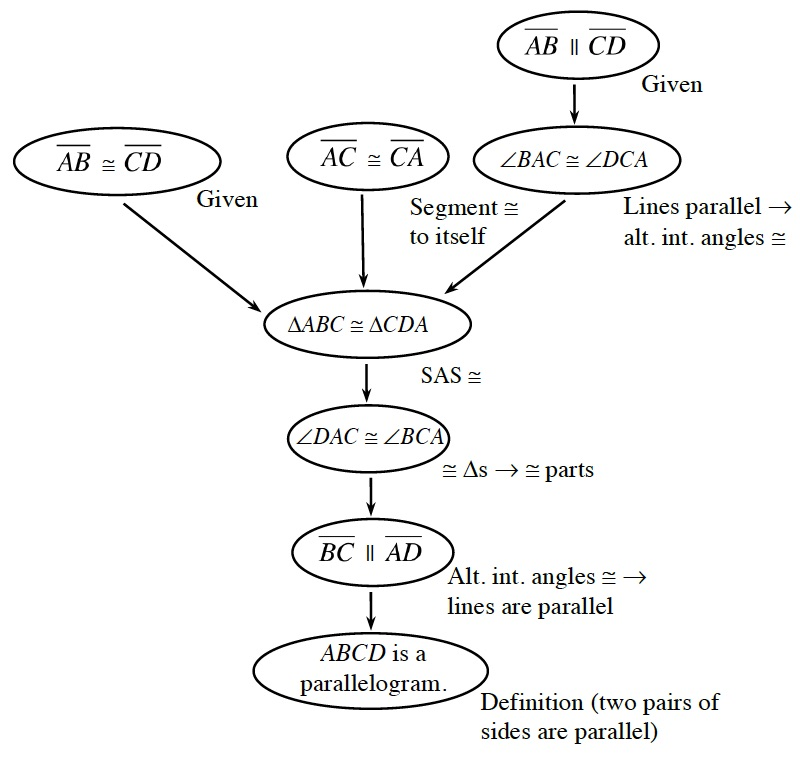### Home > GC > Chapter 7 > Lesson 7.2.5 > Problem7-85

7-85.What else can you prove about parallelograms? Prove that if a pair of opposite sides of a quadrilateral are congruent and parallel, then the quadrilateral must be a parallelogram. For example, for the quadrilateral $ABCD$ below, given that $\overline{AB}//\overline{CD}$ and $\overline{AB}≅\overline{CD}$, show that $\overline{BC}//\overline{AD}$. Organize your reasoning in a flowchart. Then record your theorem in your Theorem Toolkit (Lesson 7.2.1A Resource Page). Homework Help ✎Be sure to supply the reasoning for each step.

If a pair of opposite sides is parallel and congruent then the quadrilateral is a parallelogram.
Use the if part of the statement as the givens, and the then part of the statement as the prove portion in the proof.